In the name of ALLAH, the most beneficient, the most merciful

# Solved Examples (Quantitative Comparison)

Directions: You are given two quantities, one in column A and one in column B. You are to compare the two quantities and choose one of the followings:

A: if the quantity in Column A is greater;
B: if the quantity in Column B is greater;
C: if the two quantities are equal;
D: if the comparison cannot be determined from the information given.

1. Column A Column B
4.78498 4 3⁄4
A. Because 4 3⁄4 is equivalent to 4.75, Column A is greater.
2. Column A Column B
9/11 11/13
B. Converting each fraction to a decimal (dividing numerator by denominator) gives .82 for Column A and .84 for Column B. A faster way to compare two fractions is to crossmultiply up: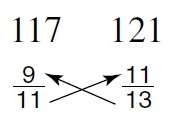The larger product (121) is above Column B, so Column B is greater.
3. Column A Column B
x > 0
x + x/2 x - x/2
A. First eliminate the equal values of x from each side, leaving (x/2) in Column A and –(x/2) in Column B. The information centered between the columns indicates that x is positive. Plugging in any positive value for x will result in Column A being positive and Column B being negative. So Column A is greater.
4. Column A Column B∠ABC + ∠BAC ∠CDB
A. Since ΔABC is an equilateral triangle, ∠ABC and ∠BAC = 60°. So Column A = 120°.Looking at ΔCDB, ∠CDB must be less than 120° because ∠BCD already equals 60° and there is still another angle (CBD) in ΔCDB.
5. Column A Column BC. The definition of median is that it divides the side it intersects into two equal parts.
6. Column A Column BAB + BD BC
A. Since Δ ABC is equilateral, AB = BC. Thus AB + BD must be more than BC alone.
7. Column A Column B
x + y = 0
x y
D. As the only condition for plugging in values for x and y is that together they must equal 0, the values for x and y may vary. For instance, both x and y may equal 0, in which case the answer would be C. Or x may be 1 and y may be –1, in which case Column A would be greater. Thus the answer is D.
8. Column A Column B
p = ± 3
(p + 2)2 26
B. Plugging in each value for p in column A, if p = +3, then (3 + 2)2 = (5)2 = 25. Plugging in –3 for p gives (–3 + 2)2 = (–1)2 = 1. In either case, Column B, 26, is greater.
9. Column A Column B
76.088 76.10
B. The only difference in the two numbers occurs after the decimal points, where .10 (Column B) is greater than .088 (Column A).
10. Column A Column B
1/19 - 1 1/18 - 1
B. In both columns, the same number, 1, is being subtracted. Therefore, the column that is greater can be determined simply by comparing the "starting" values. The column with the larger "starting" value (the number being subtracted from) will yield the larger remainder. Since 1⁄18 is larger than 1⁄19, Column B is greater. (That both remainders are negative does not affect the relationship.)
11. Column A Column B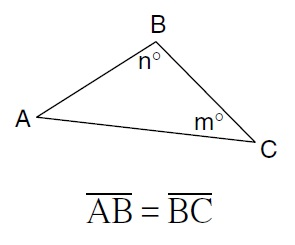n m
D. Since AB = BC,∠A = ∠C. But no information is given for ∠B. So no relationship can be determined between n and m.12. Column A Column B
53 27
B. 53 = 5 × 5 × 5 = 125, and 27 = 2 × 2 × 2 × 2 × 2 × 2 × 2 = 128.
13. Column A Column B
6x + 18y = 12
x + 3y 2
C. The information centered between the columns shows that 6x + 18y = 12. Notice that the quantity in Column A, x + 3y, exactly equals 1⁄6 of 6x + 18y. Therefore, Column A equals 1⁄6 of 12, or 2. Since Column B equals 2, the columns are equal.
14. Column A Column B
x + y = 4
xy = 0
x y
D. For xy to equal 0, either x or y must be 0. If x = 0, then y = 4. But if y = 0, then x = 4. There is no way of knowing which is which.
15. Column A Column BAB BC
D. The length of side AB is determinable by using the Pythagorean theorem, but since DC is not known, BC cannot be determined. Note that you cannot make a determination by measuring.
16. Column A Column BC. ∠BAD = ∠ABD, since angles across from equal sides in a triangle are equal.
17. Column A Column B∠DBC + ∠BCD 90o
C. Since there are 180° in a triangle and ∠BDC is 90°, the remaining two angles, ∠DBC and ∠BCD, must total 90°.
18. Column A Column BAB + BC AC
A. AB + BC is greater than AC, since the sum of any two sides of a triangle is greater than the third side.
19. Column A Column Bx y
C. In the triangle, one angle is 60°. Therefore, the remaining two angles must sum to 120° (since the total degree measure in any triangle is 180°). Since the two angles are each x, they then are equal, and each is 60°. y is the vertical angle of 60°. Since vertical angles are equal, y also equals 60°. So x = y.
20. Column A Column B
a = b
a < c
2a b + c

B. If a = b and a < c, then the following substitutions make the comparison simpler.

 2a b + c a + a b + c

Since a = b, then

 a + b b + c

Now canceling b’s from each column leaves a < c.

21. Column A Column B
a/6 = b/4
2a 3b
C. To solve a/6 = b/4
cross-multiply, giving 4a = 6b
then divide by 2
leaving 2a = 3b
22. Column A Column B
a > 0
5ab 10ab
D. The value for b could be 0, which would make Column A equal to Column B. Or b could be positive, which would make Column B greater than Column A. No relationship can be determined.
23. Column A Column B
Area of circle with diameter 8 Area of square with side 7
A. Area of circle with diameter 8 is computed by finding the radius, which is half the diameter, and substituting into this equation A = πr2. Since the radius is 4, and π is about 3.14
 π(4)2 Area of square with side 7 is 49 3.14 × 16 50.24
24. Column A Column B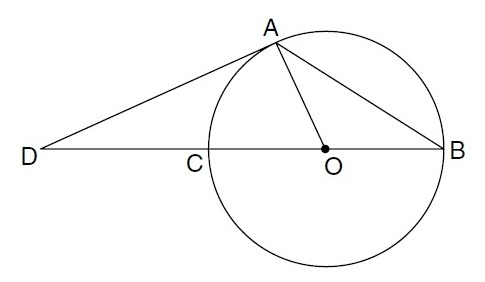arc(AC) 2(∠B)
C. arc(AC) = 2∠B, since an inscribed angle is half of the arc is subtends (connects to).
25. Column A Column BA. Since ∠AOB is a central angle, it equals the measure of arc(AB), and since ∠ADC is outside the circle but also intersects the circle at arc(AB), it is less than half of arc(AB). Therefore, ∠AOB > ∠ADC
Alternate method: The external angle AOB must be larger than either of the remote interior angles.
26. Column A Column BValue of point Q Value of point 2 1⁄3 away from point P
D. The value of point Q is 1 1⁄3. But the value of the point 2 1⁄3 away from Q may be either –2 2⁄3 or 2. So it could be either greater or less than Q. No relationship can be determined.27. Column A Column Bx y
B. The coordinates of point P are (x, y.) Since the x coordinate is to the left of the origin, x is negative. The y coordinate is above the origin, so y is positive. Therefore, Column B is greater than Column A.
28. Column A Column B
Circle O has radius 1 unit
Number of units in area of circle O Number of units in circumference of circle O
B. The area of a circle = πr2. So Column A = π(12) = π. The circumference of a circle = 2πr. So Column B = 2(π)1 = 2π.
29. Column A Column BAB arc(AB)
B. The shortest distance between two points is a straight line. Therefore, arc AB must be greater than line segment AB.30. Column A Column B
2.2 pounds in 1 kilogram
Number of kilograms in 50 pounds Number of pounds in 50 kilograms
B. Column A = 50 ÷ 2.2, and Column B = 50 × 2.2.
31. Column A Column B
T > x
y < m
x < y
x + y T + m
B. Since T is greater than x, and m is greater than y, then T + m must always be greater than x + y.
32. Column A Column BAB BC
D. In a triangle, equal angles have equal opposite sides. Therefore, AB = AC. But no information is given about angle y, and so no relationship can be drawn regarding side BC.33. Column A Column B
abc > 0
c(a + b) abc
D. Since abc > 0, you could start by plugging in 1 for each of a, b, and c. So Column A will equal c(a + b) = 1(1 + 1) = 2; Column B will equal abc = (1)(1)(1) = 1. So Column A is greater. Now plug in a different set of numbers such that abc > 0, for example, 10 for each of a, b, and c. Now Column A will equal c(a + b) = 10(10 + 10) = 200; Column B equals abc = (10)(10)(10) = 1000. Now Column B is greater. Since we find two different relationships when we use different values, no definite relationship can be determined.
34. Column A Column B
0 < a < b < c < d
c/d b/a
B. Because a, b, c, and d are each greater than 0, they are therefore positive. In Column A, the denominator is greater than the numerator, so the fraction equals less than 1. In Column B, the numerator is greater than the denominator, so the fraction equals more than 1. Therefore, Column B is greater.
35. Column A Column B
y2 + 25 (y – 5)(y – 5)
D. If y is 0, columns A and B each equal 25, and so the columns could be equal. However, if y is 1, then Column A equals 26 and Column B equals 16. No definite relationship can be determined.
36. Column A Column Bx y
D. Since ABCD is rhombus, all sides are equal. Therefore, x = (xy)/3. Solving, first crossmultiply:
3x = xy
Canceling x’s from each side: y = 3. However, knowing that y equals 3 tells nothing about the value of x.37. Column A Column B
m2 – 5m – 24 = 0
10 m
A. First factor:
m2 – 5m – 24 = 0
(m − 8)(m + 3) = 0
Now set each equal to 0:
m − 8 = 0
m = 8
m + 3 = 0
m = −3
Since both 8 and −3 are less than 10, Column A is greater.
38. Column A Column B
Number of degrees in the interior angles of a pentagon 500°
A. To find the number of degrees in the interior angles of a pentagon, use the formula 180 × (n – 2), where n is the number of sides. Therefore, 180 × (5 – 2) = 180 × 3 = 540.
540° > 500°
Another method would be to draw the pentagon and break it into triangles connecting vertices (lines cannot cross), as shown below.Multiplying the number of triangles (3) by 180 (degrees in a triangle) gives the same result, 540°.
39. Column A Column B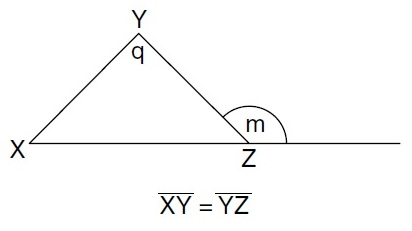q 2m – 180
C. Because XY = YZ, their opposite angles are equal. Let’s call them each x:Plugging in any value for x, say 40°, then
q = 100°
m = 140°
Therefore, Column A = 100° and Column B = 280° – 180° = 100°. The columns are equal.
40. Column A Column Bx y
C. Since DE || AB, △DCE is similar to △ACB. Therefore, because AB is 50% greater than DE, CB is 50% greater than CE, y must equal 4. Similarly, AC is 50% greater than DC, so DC must equal 4. So x = y.41. Column A Column B
Circumference of circular region C diameter d Perimeter of rectangular with region R with length 2d and width d
B. In Column A, the circumference of a circle = πd, or slightly greater than 3d (since π ≅ 3.14). In Column B, the perimeter of rectangle =
2l + 2w = 2(2d) + 2(d) = 6d
So Column B is greater.
42. Column A Column B
30¢ per pound tea X and 40¢ per pound tea Y were mixed to give 10 pounds of tea costing $3.60 Number of pounds of tea X Number of pounds of tea Y B. Since$3.60 is closer to \$4.00, there must have been more 40¢ tea. Or let x equal the number of pounds of tea X, and 10 – x equal the number of pounds of tea Y. Then
30x + 40(10 – x) = 360
30x + 400 – 40x = 360
–10x = –40
x = 4
So there were 4 pounds of tea X and 6 pounds of tea Y.
43. Column A Column B1/2 arc(AC) ∠AOB
C. Because AB is tangent to circle O, ∠AOB = 90°. Since the total interior degrees of any triangle is 180°, in triangle OAB, ∠AOB must equal 60°. Since COB is a straight line, ∠COA equals 120°. Since a central angle equals the amount of arc it intersects, arc(AC) also equals 120°. So 1⁄2 arc(AC) = 60°, and the columns are equal.44. Column A Column Bx y
B. Assume that the side shared by each of the triangles equals 1. Therefore, the triangle on the left, a 45° – 45° – 90° triangle, has sides in the ratio of 1: 1: 1 √2. So x = √2. The triangle on the right is a 30°– 60°– 90° triangle, which has sides in the ratio of 1 - √3 - 2. Therefore, y = 2. Column B is greater than Column A.45. Column A Column B60° ∠ACB
A. Because AB is the smallest side of the triangle, its opposite angle, ∠ACB, is the smallest angle. The smallest angle must be less than 60°, because if the smallest angle were equal to 60°, the three angles would sum to greater than 180°, which isn’t possible. So Column A is greater.46. Column A Column BArea of DEBC/Area of rectangleABCD 2/3
A. The area of DEBC is 3⁄4 the area of ABCD, or:
area DEBC/area ABCD = 3 units/ 4 units = 3/4
Column A is greater.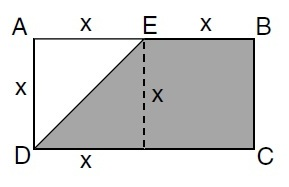47. Column A Column B5 z
A. If angle y were equal to 2x, then in the triangle, y would be 60° and x would be 30°. In a 30°–60°–90° triangle, z would be half 10, or 5. However, since y is more than twice x, x cannot be 30°; it must be less than 30°. Therefore, side z must be less than half 10, or less than 5.48. Column A Column Barc(AB) arc(AE)
A. We have no way of knowing what the measures of the angles of the circle ∠ACB or ∠ACE) are. Thus we cannot know the values of arc(AE) or arc(AB)